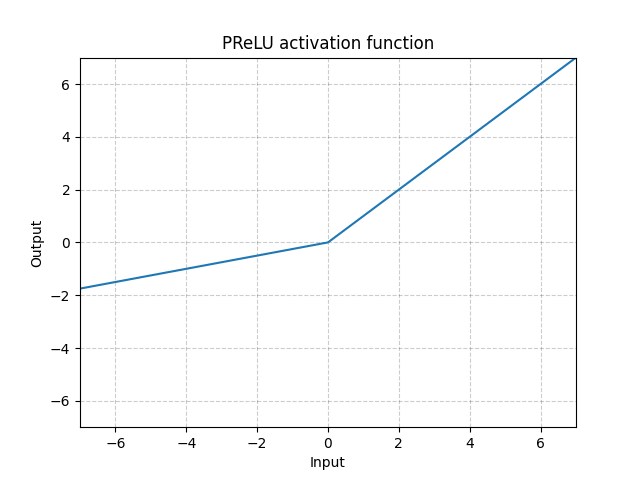/PyTorch

# PReLU

class torch.nn.PReLU(num_parameters: int = 1, init: float = 0.25) [source]

Applies the element-wise function:

$\text{PReLU}(x) = \max(0,x) + a * \min(0,x)$

or

$\text{PReLU}(x) = \begin{cases} x, & \text{ if } x \geq 0 \\ ax, & \text{ otherwise } \end{cases}$

Here $a$ is a learnable parameter. When called without arguments, nn.PReLU() uses a single parameter $a$ across all input channels. If called with nn.PReLU(nChannels), a separate $a$ is used for each input channel.

Note

weight decay should not be used when learning $a$ for good performance.

Note

Channel dim is the 2nd dim of input. When input has dims < 2, then there is no channel dim and the number of channels = 1.

Parameters
• num_parameters (int) – number of $a$ to learn. Although it takes an int as input, there is only two values are legitimate: 1, or the number of channels at input. Default: 1
• init (float) – the initial value of $a$ . Default: 0.25
Shape:
• Input: $(N, *)$ where * means, any number of additional dimensions
• Output: $(N, *)$ , same shape as the input
Variables

~PReLU.weight (Tensor) – the learnable weights of shape (num_parameters).Examples:

>>> m = nn.PReLU()
>>> input = torch.randn(2)
>>> output = m(input)


© 2019 Torch Contributors
Licensed under the 3-clause BSD License.
https://pytorch.org/docs/1.7.0/generated/torch.nn.PReLU.html